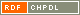# Show document

Title: Decomposition of skew-morphisms of cyclic groups Kovács, István (Author)Nedela, Roman (Author)http://amc.imfm.si/index.php/amc/article/view/157/139 English Not categorized 1.01 - Original Scientific Article IAM - Andrej Marušič Institute A skew-morphism of a group ▫$H$▫ is a permutation ▫$\sigma$▫ of its elements fixing the identity such that for every ▫$x, y \in H$▫ there exists an integer ▫$k$▫ such that ▫$\sigma (xy) = \sigma (x)\sigma k(y)$▫. It follows that group automorphisms are particular skew-morphisms. Skew-morphisms appear naturally in investigations of maps on surfaces with high degree of symmetry, namely, they are closely related to regular Cayley maps and to regular embeddings of the complete bipartite graphs. The aim of this paper is to investigate skew-morphisms of cyclic groups in the context of the associated Schur rings. We prove the following decomposition theorem about skew-morphisms of cyclic groups ▫$\mathbb Z_n$▫: if ▫$n = n_{1}n_{2}$▫ such that ▫$(n_{1}n_{2}) = 1$▫, and ▫$(n_{1}, \varphi (n_{2})) = (\varphi (n_{1}), n_{2}) = 1$▫ (▫$\varphi$▫ denotes Euler's function) then all skew-morphisms ▫$\sigma$▫ of ▫$\mathbb Z_n$▫ are obtained as ▫$\sigma = \sigma_1 \times \sigma_2$▫, where ▫$\sigma_i$▫ are skew-morphisms of ▫$\mathbb Z_{n_i}, \; i = 1, 2$▫. As a consequence we obtain the following result: All skew-morphisms of ▫$\mathbb Z_n$▫ are automorphisms of ▫$\mathbb Z_n$▫ if and only if ▫$n = 4$▫ or ▫$(n, \varphi(n)) = 1$▫. cyclic group, permutation group, skew-morphism, Schur ring 2011 str. 329-349 Vol. 4, no. 2 1855-3966 519.1 10243694921935 55Document is not linked to any category.

Average score: (0 votes) Voting is allowed only to logged in users.Hover the mouse pointer over a document title to show the abstract or click on the title to get all document metadata.

## Secondary language

Language: English ciklična grupa, permutacijska grupa, poševni morfizem, Schurov kolobar